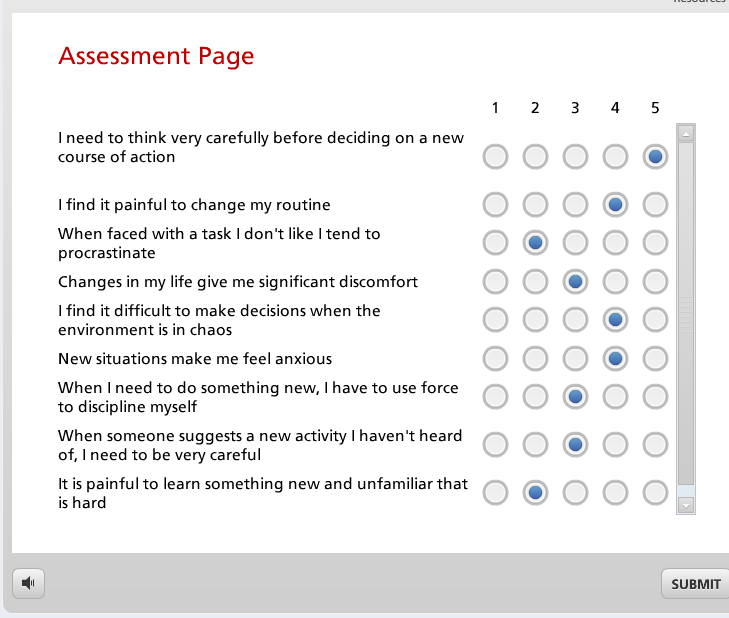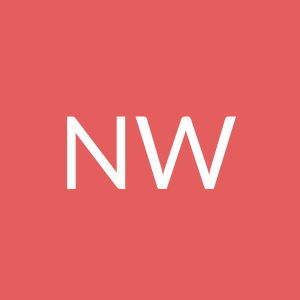# Quiz, Survey, or Javascript for one slide multiple-multiple radio

Hi,

I'm creating a one-slide "survey" with multiple questions (16 of them), each w/ multiple radio buttons to rate each question. Each answer needs to have a number associated with it and have them all added up to then take them to the appropriate slide when they're done. Here's a screenshot of what I'm trying to create:For example, if they click on a radio button "strongly agree" then that answer would = +5, and then let's say they answer "agree" on 2nd question (again, all q's on the same slide), then that answer would - 2, etc .... then at the end of the survey their #'s would be all added for a final value. That final value will then take them to the appropriate feedback slide (if their total is between 20-50, then slide 1, if 0-20, then slide 2, etc)

I can make it look like I want (multiple q's on one slide) using the Likeurt scale survey slide ... but unfortunately since it's a survey slide, I can't associate a value with each answer. I'd do it using "quiz" slides but apparently I can only have one question per slide? Is there a work around?

Is my only option to create this using javascript or is there some trick to making this all work?

Thanks!

Carl